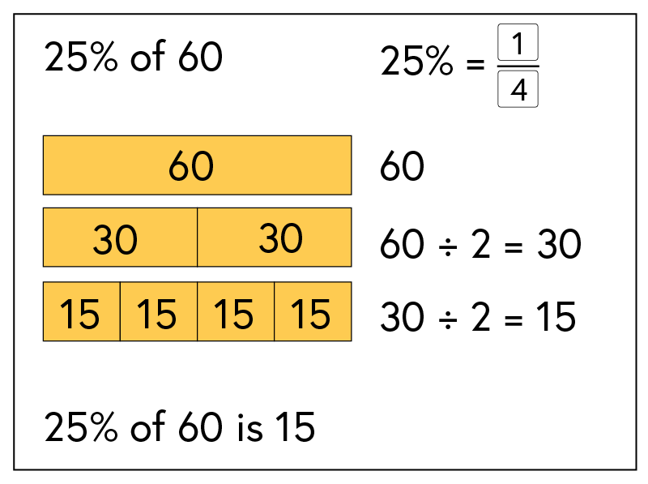## Want to keep learning?

This content is taken from the National STEM Learning Centre's online course, Maths Subject Knowledge: Fractions, Decimals, and Percentages. Join the course to learn more.
3.10

# Finding a percentage of an amount

If we want to find 25% of 60, this is the same as finding a quarter of 60. Finding a quarter is relatively simple: we halve and then halve again.

Half of 60 is 30. Half of 30 is 15.

25% of 60 = 15.